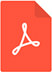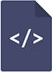# Optimization problem of attitude control of a spacecraft with bounded rotary energy using quaternionsPDF Full Text

# Abstract

The original control problem of optimal reorientation from a state of rest to a state of rest is considered and solved. The control function is torque vector. Problem of optimal control is investigated in detail for statement when control is restricted and the used functional of optimality includes kinematical rotary energy and time of maneuver. For solving and synthesis of the optimal control program, the quaternion method and the Pontryagin’s maximum principle are applied. Analytic solution of the proposed problem is presented basing on the differential equation connecting the angular velocity vector and quaternion of spacecraft attitude. It is shown that a chosen criterion of optimality provides a turn of a spacecraft with rotation energy which does not exceed the required value. This property of proposed control increases safety of flight. The time-optimal problem was solved also. The control law is formulated in the form of an explicit dependence of the control variables on the phase coordinates. All key expressions and equations are written in quaternion form which is convenient for onboard realization and implementation. The analysis of the special control regime of the spacecraft was made. Analytical formulas were written for duration of acceleration and braking. For specific cases of spacecraft configurations (dynamically symmetric spacecraft and spheric-symmetrical spacecraft as particular cases), complete solution of optimal control problem in closed form is given. Numerical example and the results of mathematical simulation for spacecraft motion under optimal control are demonstrated. This data supplements the made theoretical descriptions, and illustrates the practical feasibility of the designed algorithm for controlling the spatial orientation of the spacecraft showing reorientation process in visual form.

# Keywords

attitude, quaternion, reorientation, optimal control, criterion of optimality, maximum principle